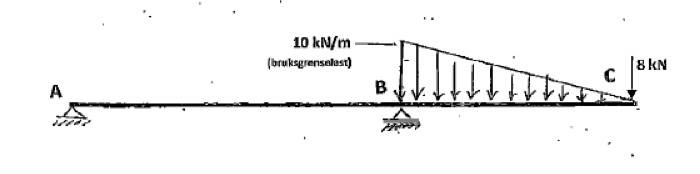# How do I find deflection in this beam?

• raymanmusic

#### raymanmusic

How do I find deflection (displacement) in point C at the end of the beam? Answer is to be given in mm. There is a roller connection (only Fy) in B and an external pin in A (Fy and Fx). The module of elasticity and area moment of inertia is given.Relevant equations:
1. http://en.wikipedia.org/wiki/Deflection_(engineering)

Here is a solution to a similar problem: https://dl.dropboxusercontent.com/u/11241083/beer_mecanica_4e_solucionario_c09_a.pdf [Broken]

Last edited by a moderator:
•1 person
First, you are going to need to know E, I, and LAB and LBC.

I tried solving this using the unit load method.
I = 57.9 * 10^6 (mm^4)
E = 2.1 * 10^5 (N/mm^2)
AB distance = 3 meters
BC distance = 2 meters

https://dl.dropboxusercontent.com/u/11241083/Solution_Beam_Problem.pdf [Broken]

Last edited by a moderator:
raymanmusic: I currently got an answer different from your post 3 answer for deflection of point C, using a different method.

Last edited by a moderator:
How do I find deflection (displacement) in point C at the end of the beam? Answer is to be given in mm. There is a roller connection (only Fy) in B and an external pin in A (Fy and Fx). The module of elasticity and area moment of inertia is given.Relevant equations:
1. http://en.wikipedia.org/wiki/Deflection_(engineering)

Here is a solution to a similar problem: https://dl.dropboxusercontent.com/u/11241083/beer_mecanica_4e_solucionario_c09_a.pdf [Broken]
You failed to use the template which was provided. Do not do this again, we have rules for a reason and they must be followed.

#### Attachments

Last edited by a moderator:
raymanmusic: I now also used a second method, and I got exactly the same answer I got using a completely different method in post 4. Therefore, I am about 99 % certain I have the correct answer.

You did excellent work in post 3, but it appears you made one main mistake, as follows. Delete your unit load at x2 = 666,67 mm, and then try your solution again. You do not want to find deflection at x2 = 666,67 mm, and you therefore should not place a unit load at x2 = 666,67 mm. Try again.

By the way, always maintain four significant digits throughout all your intermediate calculations, then round only the final answer to three significant digits. E.g., -7,556 kN, not -7,56 kN.

Thanks for helping me out nvn. Do I place the unit load where I want to find deflection? What about the triangle load? Should I ignore it when I calculate mx2? What did you get as your final answer in mm?

raymanmusic: Yes, place the unit load where you want to find deflection. Yes, ignore the triangular load when you compute mx1 and mx2. I will let you know if you obtain the correct final answer, if this is not a test question.

Last edited:
I got approximately 5.922 mm as my final answer. Is this close to the correct answer?

Excellent work, raymanmusic. Your answer is correct (5.921 54, which rounds to 5.92 mm).

By the way, N/mm^2 is called MPa. If a derived unit has a standardized special name, then only the standardized special name should be used. E.g., 210 000 MPa, not 210 000 N/mm^2.

Last edited:
•1 person
Thanks for the help nvn. I'll ask you if I have more problems I'm stuck with.

deflection101 came with a formula for this case,Where is it?

Yc=[wb^3(5L-2b)+30PLb^2] / 90

given: w=10kn/m
p=8kn/m
a= 3
b=2
EI=1 (to simplify)

Ans.:yc= - 72 (-160/3 - 56/3)

Last edited:
it doesn't get any better than this! yc= - 72

EIy(x)=wx/360b(3x^4-15bx^3+30b^2X^2-30b^3X-20ab^3)+Px/6(x^2-3bx-2ab)
EIy(2)=-72